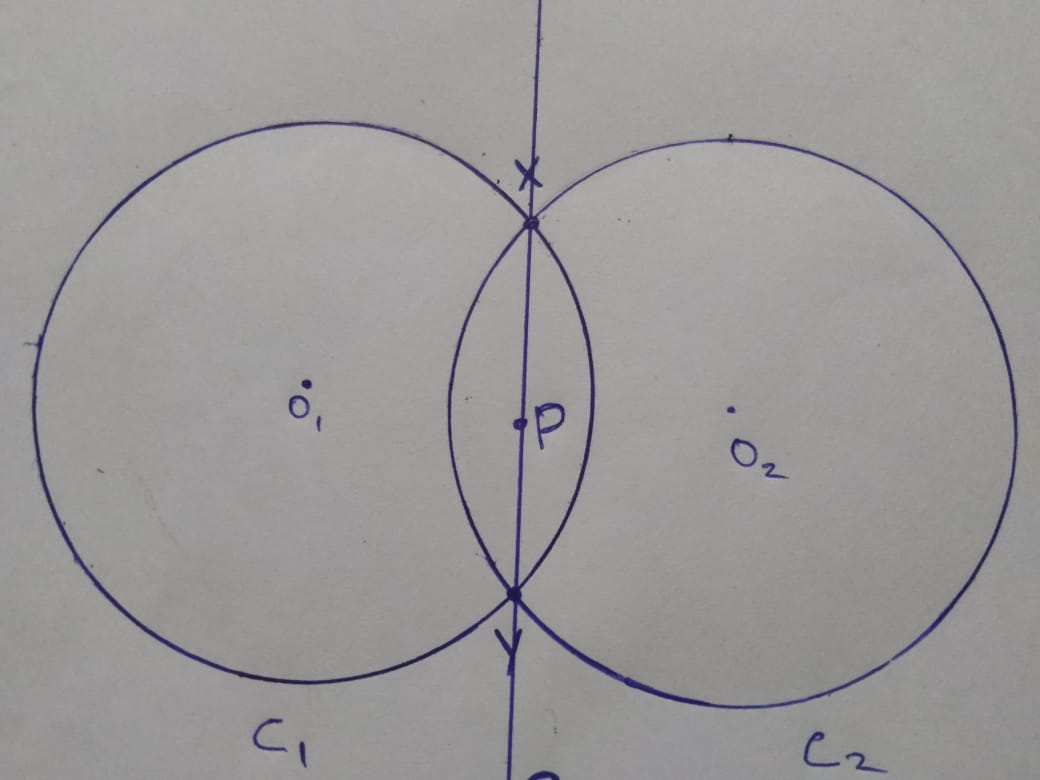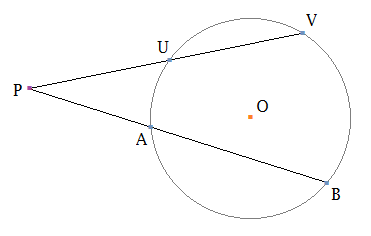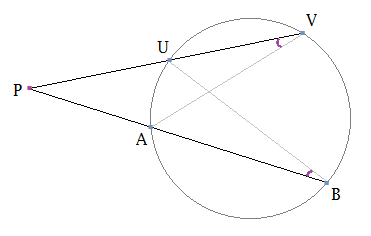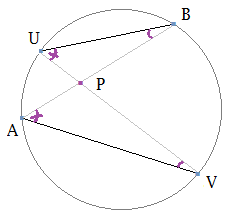In: Math

# . Let two circles C1 and C2 intersect at X and Y. Prove that a point...

. Let two circles C1 and C2 intersect at X and Y. Prove that a point P is on the line XY if and only if the power of P with respect to C1 is equal to the power of P with respect to C

## Solutions

##### Expert Solution

The power of a point theorem, due to Jakob Steiner, states that for any line through A intersecting a circle c in points P and Q, the power of the point with respect to the circle c is given up to a sign by the product

AP.AQ

of the lengths of the segments from A to P and A to Q, with a positive sign if A is outside the circle and a negative sign otherwise: if A is on the circle, the product is zero. In the limiting case, when the line is tangent to the circle, P = Q, and the result is immediate from the Pythagorean theorem.Power of p with respect to c1 =(PO1)²-r²

=PX.PY ( By the definition of

Power of the point)

Power of p with respect to c2= (PO2)²-r²

=PX.PY

PO = distance between point p and center of circle.

Therefore power of P w.r.t to c1= power of P w.r.t to c2

That implies  a point P is on the line XY if and only if the power of P with respect to C1 is equal to the power of P with respect to C.

For your understanding of power of a point
Suppose on a plane we have a point P and a circle (O). Draw a line through P which intersects with the circle at two points U and V. Then the value of

PU×PV

is independent of the choice of the line PUV.This means that if we draw another line through P which cuts the circle at two other points A and B then

PA×PB=PU×PV.

This constant value is called the power of the point P with respect to the circle (O).

To prove that PU×PV is independent of the position of the line, we will use similar triangles. We will consider two separate cases: when the point P is outside the circle and when the point P is inside the circle.

When P is outside the circle (O)We consider two triangles PUB and PAV. These two triangles are similar triangles because they have two pairs of equal angles. Thus,

PU/PA=PB/PV

From here we obtain the required equality

PA×PB=PU×PV.

When P is inside the circle (O)When P is inside the circle, we also consider the two triangles PUB and PAV. These two triangles are similar triangles because they have equal angles. So

PU/PA=PB/PV.

This implies the constancy of the power of the point P:

PA×PB=PU×PV

Also, another definition way to calculate

Power of the point = PO2​​​​ - r2

where PO is the distance between the point p and the centre of circle o, and r is the radius of the circle

## Related Solutions

##### Let R[x, y] be the set of polynomials in two coefficients. Prove that R[x, y] is...
Let R[x, y] be the set of polynomials in two coefficients. Prove that R[x, y] is a vector space over R. A polynomial f(x, y) is called degree d homogenous polynomial if the combined degree in x and y of each term is d. Let Vd be the set of degree d homogenous polynomials from R[x, y]. Is Vd a subspace of R[x, y]? Prove your answer.
##### Let C1 be the part of the exponential curve y = πe^x where 0 ≤ x...
Let C1 be the part of the exponential curve y = πe^x where 0 ≤ x ≤ 1. Let C2 be the line segment between (1, πe) and (π, 2π). If C is the union of these two curves, oriented from left to right, find the work done by the force field F = <sin(x)e^ cos(x) +y 2 , 2xy−2 sin(y) cos(y)> as a particle moves along C
##### Let f(x, y) = − cos(x + y2 ) and let a be the point a...
Let f(x, y) = − cos(x + y2 ) and let a be the point a = ( π/2, 0). (a) Find the direction in which f increases most quickly at the point a. (b) Find the directional derivative Duf(a) of f at a in the direction u = (−5/13 , 12/13) . (c) Use Taylor’s formula to calculate a quadratic approximation to f at a.
##### y = c1 cos(5x) + c2 sin(5x) is a two-parameter family of solutions of the second-order...
y = c1 cos(5x) + c2 sin(5x) is a two-parameter family of solutions of the second-order DE y'' + 25y = 0. If possible, find a solution of the differential equation that satisfies the given side conditions. The conditions specified at two different points are called boundary conditions. (If not possible, enter IMPOSSIBLE.) y(0) = 1, y'(π) = 7 y =
##### . If it has the following solution: y= c1 + c2 cos (2x) + c3 sin...
. If it has the following solution: y= c1 + c2 cos (2x) + c3 sin (2x) + c4 e3x Then:
##### Let x and y be integers such that 17 | (3x +5y). Prove that 17 |...
Let x and y be integers such that 17 | (3x +5y). Prove that 17 | (8x + 19y).
##### Two capacitors, C1 and C2, are connected in series and a battery, providing a voltage V,...
Two capacitors, C1 and C2, are connected in series and a battery, providing a voltage V, is connected across the two capacitors. (a) Find the equivalent capacitance, the energy stored in this equivalent capacitance, and the energy stored in each capacitor. (b) Show that the sum of the energy stored in each capacitor is the same as the energy stored in the equivalent capacitor. Will this equality always be true, or does it depend on the number of capacitors and...
##### 2. Prove the following properties. (b) Prove that x + ¯ xy = x + y.
2. Prove the following properties.(b) Prove that x + ¯ xy = x + y.3. Consider the following Boolean function: F = x¯ y + xy¯ z + xyz(a) Draw a circuit diagram to obtain the output F. (b) Use the Boolean algebra theorems to simplify the output function F into the minimum number of input literals.
##### Two capacitors, C1 = 28.0 µF and C2 = 40.0 µF, are connected in series, and a 21.0 V battery...
Two capacitors, C1 = 28.0 µF and C2 = 40.0 µF, are connected in series, and a 21.0 V battery is connected across them.(a) Find the equivalent capacitance, and the energy contained in this equivalent capacitor.equivalent capacitance = ?total energy stored = ?(b) Find the energy stored in each individual capacitor.energy stored in C1 = ?energy stored in C2 = ?Show that the sum of these two energies is the same as the energy found in part (a). Will this...
##### Prove that The OR operation is closed for all x, y ∈ B x + y ∈ B
Boolean AlgebraProve that The OR operation is closed for all x, y ∈ B x + y ∈ BandProve that The And operation is closed for all x, y ∈ B x . y ∈ B